# US6751030B2 - Zoom lens and image-taking apparatus - Google Patents

## Info

Publication number
US6751030B2
US6751030B2 US10/452,029 US45202903A US6751030B2 US 6751030 B2 US6751030 B2 US 6751030B2 US 45202903 A US45202903 A US 45202903A US 6751030 B2 US6751030 B2 US 6751030B2
Authority
US
United States
Prior art keywords
lens
lens unit
zoom
zoom lens
wide angle
Prior art date
Legal status (The legal status is an assumption and is not a legal conclusion. Google has not performed a legal analysis and makes no representation as to the accuracy of the status listed.)
Active
Application number
US10/452,029
Other versions
US20030227691A1 (en
Inventor
Hiroshi Saruwatari
Current Assignee (The listed assignees may be inaccurate. Google has not performed a legal analysis and makes no representation or warranty as to the accuracy of the list.)
Canon Inc
Original Assignee
Canon Inc
Priority date (The priority date is an assumption and is not a legal conclusion. Google has not performed a legal analysis and makes no representation as to the accuracy of the date listed.)
Filing date
Publication date
Priority to JP2002-163472 priority Critical
Priority to JP2002163473A priority patent/JP3927866B2/en
Priority to JP163473/2002(PAT. priority
Priority to JP2002-163473 priority
Priority to JP2002163472A priority patent/JP3977150B2/en
Application filed by Canon Inc filed Critical Canon Inc
Assigned to CANON KABUSHIKI KAISHA reassignment CANON KABUSHIKI KAISHA ASSIGNMENT OF ASSIGNORS INTEREST (SEE DOCUMENT FOR DETAILS). Assignors: SARUWATARI, HIROSHI
Publication of US20030227691A1 publication Critical patent/US20030227691A1/en
Application granted granted Critical
Publication of US6751030B2 publication Critical patent/US6751030B2/en
Active legal-status Critical Current
Anticipated expiration legal-status Critical

• 230000003287 optical Effects 0.000 claims abstract description 95
• 230000003247 decreasing Effects 0.000 claims abstract 2
• 238000006243 chemical reaction Methods 0.000 claims description 4
• 230000002349 favourable Effects 0.000 abstract description 8
• 230000014509 gene expression Effects 0.000 description 26
• 230000004075 alteration Effects 0.000 description 22
• 210000001747 Pupil Anatomy 0.000 description 5
• 230000000875 corresponding Effects 0.000 description 4
• 239000011521 glass Substances 0.000 description 2
• 206010018987 Haemorrhage Diseases 0.000 description 1
• 230000000740 bleeding Effects 0.000 description 1
• 231100000319 bleeding Toxicity 0.000 description 1
• 230000002708 enhancing Effects 0.000 description 1
• 238000003384 imaging method Methods 0.000 description 1
• 239000000463 material Substances 0.000 description 1
• 230000004048 modification Effects 0.000 description 1
• 238000006011 modification reaction Methods 0.000 description 1
• 230000001629 suppression Effects 0.000 description 1

## Images

•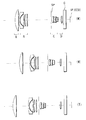•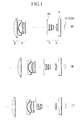•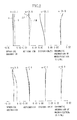•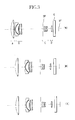•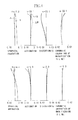•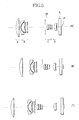•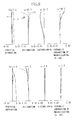•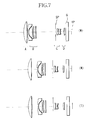•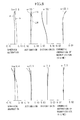•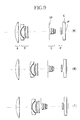•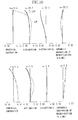•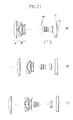•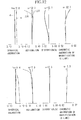•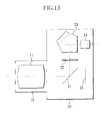## Classifications

• GPHYSICS
• G02OPTICS
• G02BOPTICAL ELEMENTS, SYSTEMS, OR APPARATUS
• G02B15/00Optical objectives with means for varying the magnification
• G02B15/14Optical objectives with means for varying the magnification by axial movement of one or more lenses or groups of lenses relative to the image plane for continuously varying the equivalent focal length of the objective
• G02B15/144Optical objectives with means for varying the magnification by axial movement of one or more lenses or groups of lenses relative to the image plane for continuously varying the equivalent focal length of the objective having four groups only
• G02B15/1441Optical objectives with means for varying the magnification by axial movement of one or more lenses or groups of lenses relative to the image plane for continuously varying the equivalent focal length of the objective having four groups only the first group being positive
• G02B15/144113Optical objectives with means for varying the magnification by axial movement of one or more lenses or groups of lenses relative to the image plane for continuously varying the equivalent focal length of the objective having four groups only the first group being positive arranged +-++
• GPHYSICS
• G02OPTICS
• G02BOPTICAL ELEMENTS, SYSTEMS, OR APPARATUS
• G02B15/00Optical objectives with means for varying the magnification
• G02B15/14Optical objectives with means for varying the magnification by axial movement of one or more lenses or groups of lenses relative to the image plane for continuously varying the equivalent focal length of the objective
• G02B15/16Optical objectives with means for varying the magnification by axial movement of one or more lenses or groups of lenses relative to the image plane for continuously varying the equivalent focal length of the objective with interdependent non-linearly related movements between one lens or lens group, and another lens or lens group
• G02B15/163Optical objectives with means for varying the magnification by axial movement of one or more lenses or groups of lenses relative to the image plane for continuously varying the equivalent focal length of the objective with interdependent non-linearly related movements between one lens or lens group, and another lens or lens group having a first movable lens or lens group and a second movable lens or lens group, both in front of a fixed lens or lens group
• G02B15/167Optical objectives with means for varying the magnification by axial movement of one or more lenses or groups of lenses relative to the image plane for continuously varying the equivalent focal length of the objective with interdependent non-linearly related movements between one lens or lens group, and another lens or lens group having a first movable lens or lens group and a second movable lens or lens group, both in front of a fixed lens or lens group having an additional fixed front lens or group of lenses
• G02B15/173Optical objectives with means for varying the magnification by axial movement of one or more lenses or groups of lenses relative to the image plane for continuously varying the equivalent focal length of the objective with interdependent non-linearly related movements between one lens or lens group, and another lens or lens group having a first movable lens or lens group and a second movable lens or lens group, both in front of a fixed lens or lens group having an additional fixed front lens or group of lenses arranged +-+

## Abstract

A zoom lens comprising four lens units is disclosed which achieves a high variable magnification ratio and favorable optical performance at an arbitrary zoom position. The zoom lens of the present invention includes, in order from an object side, a first lens unit having positive optical power, a second lens unit having a negative optical power, a third lens unit having a positive optical power, and a fourth lens unit having a positive optical power. During zooming from the wide angle end to the telephoto end, the spacing between the first and second lens units is increased, the spacing between the second and third lens units is decreased, the spacing between the third and fourth lens units is increased, and an aperture stop moves together with the third lens unit. In addition, specific conditions are satisfied.

## Description

BACKGROUND OF THE INVENTION
1. Field of the Invention
The present invention relates to a zoom lens, and more particularly, to a zoom lens which is preferable for use in an image-taking apparatus such as a video camera and a digital camera which uses a solid-state image pickup element as an image pickup device.
2. Description of the Related Art
Conventionally, a zoom lens of a so-called negative lead type having a lens unit with a negative optical power at the front has been used as a standard zoom lens in many cameras since it relatively easily achieves image taking at a wider angle.
As an exemplary standard zoom lens of this type, Japanese Patent Application Laid-Open No. 1978-132360 (corresponding to U.S. Pat. No. No.4,299,452), Japanese Patent Application Laid-Open No. 1981-19022 (corresponding to U.S. Pat. No. 4,370,031), and U.S. Pat. No. 5,283,639 each have proposed or disclosed a so-called two unit zoom lens consisting of two lens units, that is, a first lens unit having a negative optical power and a second lens unit having a positive optical power, in which these two lens units are moved along an optical axis to change the spacing between the lens units to provide zooming.
Japanese Patent Application Laid-Open No. 1995-52256 has proposed a zoom lens having, in order from an object side, a first lens unit having a negative optical power, a second lens unit having a positive optical power, and a third lens unit having a positive optical power, in which the spacing between the second lens unit and the third lens unit is increased to provide zooming from the wide angle end to the telephoto end.
In addition, U.S. Pat. No. 5,434,710 has disclosed a zoom lens having, in order from an object side, three lens units consisting of a first lens unit having a negative optical power, a second lens unit having a positive optical power, and a third lens unit having a positive optical power, in which the spacing between the second lens unit and the third lens unit is reduced to provide zooming from the wide angle end to the telephoto end.
The present inventor has also proposed a multiunit zoom lens comprising of three or more lens units in Japanese Patent Application Laid-Open No. 1994-27377 (corresponding to U.S. Pat. No. 6,104,548).
Typically, the negative lead type zoom lens having a lens unit with a negative optical power at the front is characterized in that image taking is relatively easily performed at a wider angle and that a predetermined back focal distance can be readily provided.
To achieve favorable optical performance throughout the zoom range or throughout an image plane, it is necessary to appropriately set the arrangement of the optical powers of respective lens units and the shapes of lenses.
Inappropriately setting the arrangement of the optical powers of the respective lens units and the lens shapes increases variations in aberration associated with to cause difficulty in providing high optical performance throughout the zoom range.
Especially, in the two unit zoom lens having a lens unit with a negative optical power at the front, the relative positions of the respective lens units on an optical axis are uniquely determined resulting from varied magnification and corrected variations in the position of an image plane. As a result, optical performance cannot be controlled arbitrarily at a certain position while zooming from the wide angle end to the telephoto end.
Variations in aberration need to be minimized in the respective lens units during zooming in order to provide favorable optical performance at a certain point during zooming. As a method to address this, for example, the optical power is reduced in each lens unit, or a larger number of lens elements are used to form each lens unit. These methods, however, lead to an increase in the overall length of the lenses and difficulty in achieving high zooming ratio and high performance.
To solve the problems, U.S. Pat. No. 5,570,233 has disclosed a zoom lens consisting of, in order from an object side, a first lens unit having a positive optical power, a second lens unit having a negative optical power, a third lens unit having a positive optical power, and a fourth lens unit having a positive optical power, in which each lens unit is moved to provide zooming.
With the development of the image pickup device, however, further improvement in optical performance is required in the field of optical apparatuses such as a video camera and a digital camera in which increasingly higher performance is desired.
Additionally, in a zoom lens of a type in which a lens unit closer to an image plane than an aperture stop moves in association with zooming, the exit pupil distance largely varies as a higher zooming ratio is provided. In a CCD frequently used as an image pickup device in recent years, for example, if the incident angle of light rays on an imaging plane varies, the angle of the light rays passing through a color filter is changed to create the possibility of causing a problem of color bleeding on the periphery of an image.
SUMMARY OF THE INVENTION
It is an object of the present invention to provide a zoom lens comprising four lens units which achieves a high zoom ratio and provides favorable optical performance at an arbitrary zoom position.
It is another object of the present invention to provide a zoom lens which involves small variations in the exit pupil distance during zooming, in addition to the aforementioned object.
To achieve the aforementioned objects, the zoom lens according to an aspect of the present invention includes, in order from an object side to an image side, a first lent unit which has a positive optical power, a second lens unit which has a negative optical power, a third lens unit which has a positive optical power, and a fourth lens unit which has a positive optical power. During zooming from the wide angle end to the telephoto end, the spacing between the first lens unit and the second lens unit is increased, the spacing between the second lens unit and the third lens unit is reduced, the spacing between the third lens unit and the fourth lens unit is increased, and an aperture stop moves together with the third lens unit. In addition, specific conditions described in the following embodiments are satisfied.
According to another aspect of the present invention, the zoom lens includes, in order from an object side to an image side, a first lens unit which has a positive optical power, a second lens unit which has a negative optical power, a third lens unit which has a positive optical power, and a fourth lens unit which has a positive optical power. The spacing between the respective lens units changes during zooming, and specific conditions described in the following embodiments are satisfied.
Characteristics of the zoom lens of the present invention will be apparent from the following description of specific embodiments with reference to the drawings.
BRIEF DESCRIPTION OF THE DRAWINGS
FIG. 1 is a section view of a zoom lens which is Embodiment 1 of the present invention;
FIG. 2 shows aberrations in Numerical Example 1 of the present invention;
FIG. 3 is a section view of a zoom lens which is Embodiment 2 of the present invention;
FIG. 4 shows aberrations in Numerical Example 2 of the present invention;
FIG. 5 is a section view of a zoom lens which is Embodiment 3 of the present invention;
FIG. 6 shows aberrations in Numerical Example 3 of the present invention;
FIG. 7 is a section view of a zoom lens which is Embodiment 4 of the present invention;
FIG. 8 shows aberrations in Numerical Example 4 of the present invention;
FIG. 9 is a section view of a zoom lens which is Embodiment 5 of the present invention;
FIG. 10 shows aberrations in Numerical Example 5 of the present invention;
FIG. 11 is a section view of a zoom lens which is Embodiment 6 of the present invention;
FIG. 12 shows aberrations in Numerical Example 6 of the present invention; and
FIG. 13 is a section view of a digital camera having the zoom lens of any of Embodiments 1 to 6.
DETAILED DESCRIPTION OF THE PREFERRED EMBODIMENTS
Preferred embodiments of the present invention are hereinafter described with reference to the drawings.
An image-taking apparatus which embodies the present invention uses a zoom lens according to any of Embodiment 1 to Embodiment 6 shown in FIGS. 1, 3, 5, 7, 9, and 11 to form an object image on a solid-state image pickup element (photoelectric conversion element) such as a CCD and a CMOS sensor disposed on an image plane IP.
First of all, description is made for zoom lenses according to Embodiment 1 to Embodiment 3 shown in FIGS. 1, 3, and 5.
FIGS. 1, 3, and 5 are section views showing the zoom lenses of Embodiment 1 to Embodiment 3, in which (W) represents the wide angle end, (M) an middle zoom position, and (T) the telephoto end. In each section view, in order from an object side (the left side in each figure), letter A shows a first lens unit having a positive optical power (the optical power is the reciprocal of the focal length of the lens unit), letter B a second lens unit having a negative optical power, letter C shows a third lens unit having a positive optical power, and letter D shows a fourth lens unit having a positive optical power.
SP shows an aperture stop and G shows a glass block such as a optical filter or a face plate.
In the zoom lens in each of Embodiment 1 to Embodiment 3, the entire zoom lens system comprises at least four lens units, and zooming is provided by changing the spacing between the respective units on an optical axis.
In Embodiment 1 to Embodiment 3, for zooming from the wide angle end to the telephoto end, the spacing between the first lens unit A and the second lens unit B is increased, the spacing between the second lens unit B and the third lens unit C is reduced, and the spacing between the third lens unit C and the fourth lens unit D is increased. The aperture stop SP moves together with the third lens unit C.
Each zoom lens system in Embodiment 1 to 3 satisfies the following conditional expression:
−1.0<(Cw−Ct)/f 3<−0.6  (1)
where Cw represents the spacing between the third lens unit C and the fourth lens unit D on the optical axis at the wide angle end, Ct represents the spacing between the third lens unit C and the fourth lens unit D on the optical axis at the telephoto end, and f3 represents the focal length of the third lens unit C.
The conditional expression (1) relates to the track of movement and the optical power of the third lens unit C. If the ratio of (Cw−Ct)/f3 exceeds the upper limit in the conditional expression (1), the third lens unit C moves over an unacceptably long distance, and thus variations in the exit pupil cannot be suppressed. On the other hand, if the ratio is less than the lower limit, the third lens unit C has such a low optical power that it is difficult to reduce the overall length of the zoom lens.
In Embodiment 1 to Embodiment 3, for zooming from the wide angle end to the telephoto end, the third lens unit C moves toward the object side, and the fourth lens unit D moves along a track which is convex toward the object side. The following conditional expression is preferably satisfied:
0.2<fw/f 4<0.35  (2)
where fw represents the focal length of the entire zoom lens system at the wide angle end, and f4 represents the focal length of the fourth lens unit D.
The conditional expression (2) relates to the optical power of the fourth lent unit D. If the ratio of fw/f4 exceeds the upper limit in the conditional expression (2), the refractive power of the fourth lens unit D is so high that it is impossible to eliminate the influence of variations in the aperture stop SP, which moves together with the third lens unit C, exerting upon variations in the exit pupil. On the other hand, if the ratio is less than the lower limit, the optical power of the fourth lens unit D is so low that it is difficult to suppress variations in aberration in zooming which is advantageously small in the structure of the four lens unit.
To maintain a small overall length of the zoom lens, it is preferable that the first lens unit A and the second lens unit B are moved along a track which is convex toward the image side when zooming from the wide angle end to the telephoto end. As a result, variations in aberration can be reduced throughout the entire zoom range.
In addition, the focal length of the fourth lens unit D preferably satisfies:
0.25<fw/f 4<0.35  (3)
when the third lens unit C consists of a positive lens element, a cemented lens in which a positive lens element and a negative lens element are cemented, in order from the object side.
Furthermore, the following conditional expression is preferably satisfied:
0.2<fw/f 4<0.3  (4)
when the third lens unit C consists of a positive lens element, a cemented lens in which a positive lens element and a negative lens element are cemented, and a positive lens element, in order from the object side.
More preferably, the following conditional expression is satisfied when the third lens unit C consists of a positive lens element, a cemented lens in which a positive lens element and a negative lens element are cemented, and a positive lens element, in order from the object side:
0.1<f 3/f 3 r<0.4  (5)
where f3r represents the focal length of the lens element closest to the image side of the third lens unit C.
The conditional expression (5) defines a proper range for maintaining a proper focal length of the lens closest to the image side of the third lens unit C to reduce variations in the emergent angle of light rays emerging from the third lens unit C.
Next, numerical examples of Embodiment 1 to Embodiment 3 are shown. A numerical example of Embodiment 1 shown in FIG. 1 is referred to as Numerical Example 1, a numerical example of Embodiment 2 in FIG. 3 as Numerical Example 2, and a numerical example of Embodiment 3 in FIG. 5 as Numerical Example 3.
In each numerical example, ri represents the radius of curvature of the i-th lens surface in order from the object side, di represents the thickness of the i-th optical member in order from the object side or the air space corresponding thereto, and ni and vi represent the refractive index and the Abbe number of the material of the i-th optical member in order from the object side.
When a lens surface is an aspheric shape, the aspheric shape is represented by the following expression: $X=\frac{\left(1/R\right)\text{ }{Y}^{2}}{1+\sqrt{\left(1-\left(1+K\right)\text{ }{\left(Y/R\right)}^{2}\right)}}+{\mathrm{BY}}^{4}+{\mathrm{CY}}^{6}+{\mathrm{DY}}^{8}+{\mathrm{EY}}^{10}$
where R represents the radius of curvature of the center of the lens surface, an X axis represents an optical axis direction (traveling direction of light), a Y axis represents a direction perpendicular to the optical axis, a K represents the conic constant, and B, C, and D, are aspheric coefficients. “e-X” means “x10−x.” Furthermore, in each numerical example, f represents a focal length, fno represents a F number, and ω represents half of the field angle.
Numerical Example 1
 TABLE 1 f = 7.46779  fno = 1:2.7  2ω = 63.2
 r1 = 35.389 d1 = 5.50 n1 = 1.51633 ν1 = 64.1 r2 = −528.126 d2 = VARIABLE r3 = 71.708 d3 = 1.30 n2 = 1.80400 ν2 = 46.6 r4 = 11.296 d4 = 5.50 r5 = −73.005 d5 = 1.00 n3 = 1.77250 ν3 = 49.6 r6 = 27.283 d6 = 1.70 r7 = 21.795 d7 = 2.80 n4 = 1.84666 ν4 = 23.9 r8 = 103.969 d8 = VARIABLE r9 = ∞ (APERA- d9 = 0.80 TURE STOP) r10 = 9.786 d10 = 2.80 n5 = 1.72916 ν5 = 54.7 r11 = 54.605 d11 = 0.30 r12 = 12.273 d12 = 2.40 n6 = 1.69350 ν6 = 53.2 r13 = 25.204 d13 = 0.70 n7 = 1.84666 ν7 = 23.9 r14 = 7.182 d14 = 2.20 r15 = −81.570 d15 = 1.40 n8 = 1.51633 ν8 = 64.1 r16 = −24.163 d16 = VARIABLE r17 = 28.000 d17 = 2.00 n9 = 1.80610 ν9 = 40.7 r18 = −1242.352 d18 = VARIABLE r19 = ∞ d19 = 4.00 n10 = 1.51680 ν10 = 64.2 r20 = ∞
 FOCAL LENGTH/VARIABLE SPACING 7.47 16.47 36.29
 d2 1.20 10.92 18.54 d8 37.10 15.13 3.55 d16 4.06 7.91 22.43 d18 2.00 4.36 4.91
 ASPHERIC SURFACE
 12TH SURFACE R = 12.273 K = 6.160E−1 B = −1.849E−4 C = −1.558E−6 D = −2.399E−8 17TH SURFACE R = 28.0 K = 3.407E−1 B = −1.954E−6 C = 9.748E−8 D = −3.041E−9
Numerical Example 2
 TABLE 2 f = 7.45587  fno = 1:2.8  2ω = 66.2
 r1 = 33.880 d1 = 6.00 n1 = 1.51633 ν1 = 64.1 r2 = −7025.879 d2 = VARIABLE r3 = 54.832 d3 = 1.30 n2 = 1.80400 ν2 = 46.6 r4 = 11.212 d4 = 5.93 r5 = −72.278 d5 = 1.00 n3 = 1.77250 ν3 = 49.6 r6 = 28.966 d6 = 1.70 r7 = 22.856 d7 = 2.80 n4 = 1.84666 ν4 = 23.9 r8 = 103.245 d8 = VARIABLE r9 = ∞ (APERA- d9 = 0.80 TURE STOP) r10 = 9.193 d10 = 2.80 n5 = 1.69680 ν5 = 55.5 r11 = 224.107 d11 = 0.30 r12 = 12.293 d12 = 2.40 n6 = 1.69350 ν6 = 53.2 r13 = 26.680 d13 = 0.70 n7 = 1.84666 ν7 = 23.9 r14 = 6.690 d14 = VARIABLE r15 = 20.006 d15 = 2.00 n8 = 1.80610 ν8 = 40.7 r16 = 594.211 d16 = VARIABLE r17 = ∞ d17 = 4.00 n9 = 1.51633 ν9 = 64.2 r18 = ∞
 FOCAL LENGTH/VARIABLE SPACING 7.46 16.45 36.32
 d2 1.00 10.93 20.76 d8 37.42 14.90 3.57 d14 6.73 10.17 22.87 d16 2.00 4.56 5.72
 ASPHERIC SURFACE
 12TH SURFACE R = 12.29 K = 4.853E−3 B = −1.833E−4 C = −2.283E−6 D = −3.066E−8 15TH SURFACE R = 20.01 K = 2.087 B = −4.443E−5 C = −2.156E−8 D = −5.081E−9
Numerical Example 3
 TABLE 3 f = 7.44598  fno = 1:2.8  2ω = 65.4
 r1 = 36.174 d1 = 5.50 n1 = 1.51633 ν1 = 64.2 r2 = −2716.614 d2 = VARIABLE r3 = 50.435 d3 = 1.30 n2 = 1.77250 ν2 = 49.6 r4 = 10.608 d4 = 4.70 r5 = 198.546 d5 = 1.00 n3 = 1.77250 ν3 = 49.6 r6 = 16.816 d6 = 1.70 r7 = 15.988 d7 = 2.80 n4 = 1.84666 ν4 = 23.9 r8 = 37.416 d8 = VARIABLE r9 = ∞ (APERA- d9 = 2.30 TURE STOP) r10 = 9.853 d10 = 2.80 n5 = 1.74330 ν5 = 49.3 r11 = −422.142 d11 = 0.30 r12 = 11.114 d12 = 2.40 n6 = 1.69680 ν6 = 55.5 r13 = 38.099 d13 = 0.70 n7 = 1.84666 ν7 = 23.9 r14 = 6.158 d14 = 2.00 r15 = 254.815 d15 = 1.40 n8 = 1.60311 ν8 = 60.7 r16 = −146.162 d16 = VARIABLE r17 = 16.773 d17 = 3.40 n9 = 1.73077 ν9 = 40.5 r18 = −25.584 d18 = 0.70 n10 = 1.69680 ν10 = 55.5 r19 = 96.538 d19 = VARIABLE r20 = ∞ d20 = 4.00 n11 = 1.51680 ν11 = 64.2 r21 = ∞
 FOCAL LENGTH/VARIABLE SPACING 7.45 16.61 37.00
 d2 2.00 11.42 23.20 d8 30.00 10.07 1.80 d16 3.62 6.80 19.61 d19 3.04 6.02 6.39
 ASPHERIC SURFACE
 10TH SURFACE R = 9.853 K = −2.505 B = 2.312E−4 C = −1.947E−6 D = 4.993E−8 E = −1.152E−9 17TH SURFACE R = 16.774 K = 8.657E−1 B = −3.579E−5 C = 1.114E−7 D = −1.522E−9 E = 2.917E−10
Table 4 shows the values calculated with the aforementioned conditional expressions (1) to (5) in Numerical Examples 1 to 3.
 TABLE 4 Numerical Numerical Numerical Example 1 Example 2 Example 3
 Conditional −0.84 −0.72 −0.82 expression (1) conditional 0.22 0.29 0.29 expressions (2) to (4) conditional 0.33 — 0.13 expression (5)
FIGS. 2, 4 and 6 show aberrations in Numerical Examples 1 to 3, respectively. In each of the figures, a curve d represents the aberration of a d line, a curve g represents the aberration of a g line, a curve ΔS represents the aberration on a sagittal image plane, and a curve ΔM represents the aberration on a meridional image plane, respectively.
As described above, according to Embodiment 1 to Embodiment 3, appropriately setting the arrangement of the optical powers in the respective lens units and the track of movement during zooming in the zoom lens of the four lens unit structure can provide favorable optical performance at an arbitrary zoom position and can reduce variations in the exit pupil distance throughout the entire zoom range.
Next. Embodiment 4 to Embodiment 6 are described.
FIGS. 7, 9, and 11 are section views showing lenses of zoom lenses of Embodiment 4 to Embodiment 6, in which (W) represents the wide angle end, (M) an middle zoom position, and (T) the telephoto end. In each section view, in order from an object side (the left side in each figure), letter A shows a first lens unit having a positive optical power, letter B shows a second lens unit having a negative optical power, letter C shows a third lens unit having a positive optical power, and letter D shows a fourth lens unit having a positive optical power.
SP shows an aperture stop and G shows a glass block such as an optical filter or a face plate.
In the zoom lens in each of Embodiment 4 to Embodiment 6, the entire zoom lens system comprises four lens units, and zooming is provided by changing the spacing between the respective units on an optical axis.
Especially, the moving direction of the second lens unit B is reversed to follow a convex track toward the image side while zooming from the wide angle end to the telephoto end. Thus, the spacing margin between the second lens unit B and the third lens unit C can be reduced to achieve a reduction in size of the entire zoom lens system.
In each of Embodiment 4 to Embodiment 6, the following is satisfied:
0.9<bwm/bwt<2.0  (6)
where bwm represents an amount of movement of the second lens unit B from the wide angle end to the position where the focal length fm of the entire zoom lens system is equal to the geometric mean $\left(\mathrm{fm}=\sqrt{\left(\mathrm{fw}·f\text{ }t\right)}\right)$
of the focal length fw at the wide angle end and the focal length ft at the telephoto end, and bwt represents an amount of movement of the second lens unit B from the wide angle end to the telephoto end.
The conditional expression (6) relates to the moving amount of the second lens unit B in zooming. If the ratio of bwm/bwt exceeds the upper limit in the conditional expression (6), the second lens unit B moves over a large distance in the portion of the zoom region from the wide angle end to the middle point, making it difficult to achieve high zoom ratio of the entire zoom lens system. On the other hand, if the ratio of bwm/bwt is less than the lower limit, favorable correction of aberration is difficult to achieve in the middle zoom range.
In each of Embodiment 4 to Embodiment 6, appropriate arrangement of optical powers enables improved optical performance in the middle of zooming. In addition, when the first lens unit A consists of a single positive lens element, it is possible to provide both a reduced size of the entire zoom lens system and favorable correction of aberration.
In each of Embodiment 4 to Embodiment 6, the following conditional expression needs to be satisfied to form the first lens unit A of the single positive lens element:
0.05<fw/f 1<0.15  (7)
where fw represents the focal length of the entire zoom lens system at the wide angle end, and f1 represents the focal length of the first lens unit A.
The conditional expression (7) relates to the focal length of the first lens unit A. If the ratio of fw/f1 exceeds the upper limit in the conditional expression (7), the first lens unit A has such a high power that the diameter of the first lens unit A is large. On the other hand, if the ratio is less than the lower limit, the first lens unit A disadvantageously has a low power to increase the overall length of the zoom lens required for a desired zoom ratio.
In addition, the first lens unit A is formed as a movable unit, and the overall length of the zoom lens at the telephoto end is set to be larger than the overall length of the zoom lens at the wide angle end. It is thus possible to reduce the burden of variable magnification on the other lens units.
The moving direction of the first lens unit A is reversed to follow a convex track toward the image side while zooming from the wide angle end to the telephoto end. This enables reduced variations in optical performance while zooming and a smaller diameter of the first lens unit A.
The third lens unit C is moved monotonously toward the object side (in such a manner that the moving direction is not changed) when zooming from the wide angle end to the telephoto end, and the fourth lens unit D is moved along a convex track toward the object side. Thus, optical performance can be enhanced throughout the entire zoom range in addition to a reduction in the burden of variable magnification on the second lens unit B.
To further enhance the performance, in each of Embodiment 4 to Embodiment 6, the following is preferably satisfied:
0.1<(Cw−Cm)/(Cw−Ct)<0.3  (8)
−0.9<f 2 /f 3(or f 3 /fw)<−0.6  (9)
where Cw represents the spacing between the third lens unit C and the fourth lens unit D on the optical axis at the wide angle end, Ct the spacing between the third lens unit C and the fourth lens unit D on the optical axis at the telephoto end, Cm the spacing between the third lens unit C and the fourth lens unit D on the optical axis at the focal length fm of the entire zoom lens system, and fi the focal length of the i-th lens unit.
Both of the conditional expressions (8) and (9) relate to the arrangement of the optical power of the third lens unit C, and are important to appropriate burden sharing of variable magnification between the second lens unit B and the third lens unit C to achieve higher performance.
The aperture stop SP is moved together with the third lens unit C to facilitate simplification of the structure of a lens barrel (not shown) for accommodating this zoom lens.
Next, numerical examples of Embodiment 4 to Embodiment 6 are shown. A numerical example of Embodiment 4 shown in FIG. 7 is referred to as Numerical Example 4, a numerical example of Embodiment 5 in FIG. 9 as Numerical Example 5, and a numerical example of Embodiment 6 in FIG. 11 as Numerical Example 6.
In Numerical Examples 4 to 6, the definitions of reference symbols and the expression for representing an aspheric lens surface are identical to those in Numerical Examples 1 to 3 described above.
 TABLE 5 f = 7.45587  fno = 1:2.8  2ω = 66.2
 r1 = 33.880 d1 = 6.00 n1 = 1.51633 ν1 = 64.1 r2 = −7025.879 d2 = VARIABLE r3 = 54.832 d3 = 1.30 n2 = 1.80400 ν2 = 46.6 r4 = 11.212 d4 = 5.93 r5 = −72.278 d5 = 1.00 n3 = 1.77250 ν3 = 49.6 r6 = 28.966 d6 = 1.70 r7 = 22.856 d7 = 2.80 n4 = 1.84666 ν4 = 23.9 r8 = 103.245 d8 = VARIABLE r9 = ∞ (APERA- d9 = 0.80 TURE STOP) r10 = 9.193 d10 = 2.80 n5 = 1.69680 ν5 = 55.5 r11 = 224.107 d11 = 0.30 r12 = 12.293 d12 = 2.40 n6 = 1.69350 ν6 = 53.2 r13 = 26.680 d13 = 0.70 n7 = 1.84666 ν7 = 23.9 r14 = 6.690 d14 = VARIABLE r15 = 20.006 d15 = 2.00 n8 = 1.80610 ν8 = 40.7 r16 = 594.211 d16 = VARIABLE r17 = ∞ d17 = 4.00 n9 = 1.51633 ν9 = 64.2 r18 = ∞
 FOCAL LENGTH/VARIABLE SPACING 7.46 16.45 36.32
 d2 1.00 10.93 20.76 d8 37.42 14.90 3.57 d14 6.73 10.17 22.87 d16 2.00 4.56 5.72
 ASPHERIC SURFACE
 12TH SURFACE R = 12.29 K = 4.853E−3 B = −1.833E−4 C = −2.283E−6 D = −3.066E−8 17TH SURFACE R = 20.01 K = 2.087 B = −4.443E−5 C = −2.156E−8 D = 5.081E−9
Numerical Example 5
 TABLE 6 f = 7.45587  fno = 1:2.6  2ω = 66.4
 r1 = 40.266 d1 = 6.00 n1 = 1.48749 ν1 = 70.2 r2 = −734.702 d2 = VARIABLE r3 = 76.424 d3 = 1.30 n2 = 1.80400 ν2 = 46.6 r4 = 11.861 d4 = 5.35 r5 = −67.123 d5 = 1.00 n3 = 1.77250 ν3 = 49.6 r6 = 31.704 d6 = 1.70 r7 = 23.332 d7 = 2.80 n4 = 1.84666 ν4 = 23.9 r8 = 100.885 d8 = VARIABLE r9 = ∞ (APERA- d9 = 0.80 TURE STOP) r10 = 9.818 d10 = 2.80 n5 = 1.69680 ν5 = 55.5 r11 = 87.682 d11 = 0.30 r12 = 12.726 d12 = 2.40 n6 = 1.69350 ν6 = 53.2 r13 = 20.514 d13 = 0.70 n7 = 1.84666 ν7 = 23.9 r14 = 7.404 d14 = 1.80 r15 = −66.106 d15 = 1.40 n8 = 1.51633 ν8 = 64.1 r16 = −18.575 d16 = VARIABLE r17 = 19.325 d17 = 2.00 n9 = 1.80610 ν9 = 40.7 r18 = 45.742 d18 = VARIABLE r19 = ∞ d19 = 4.00 n10 = 1.51880 ν10 = 64.2 r20 = ∞
 FOCAL LENGTH/VARIABLE SPACING 7.46 16.45 36.32
 d2 3.00 18.82 20.14 d8 39.19 19.06 3.58 d16 9.80 13.15 28.28 d18 2.00 2.96 2.00
 ASPHERIC SURFACE
 12TH SURFACE R = 12.726 K = 5.664E−1 B = −1.891E−4 C = −1.7595−6 D = −1.421E−8 17TH SURFACE R = 19.325 K = −5.355 B = 7.372E−5 C = 1.514E−6 D = −2.911E−8
Numerical Example 6
 TABLE 7 f = 7.44598  fno = 1:22.8  2ω = 65.4
 r1 = 36.74 d1 = 5.50 n1 = 1.51633 ν1 = 64.2 r2 = −2716.614 d2 = VARIABLE r3 = 50.435 d3 = 1.30 n2 = 1.77250 ν2 = 49.6 r4 = 10.608 d4 = 4.70 r5 = 198.546 d5 = 1.00 n3 = 1.77250 ν3 = 49.6 r6 = 16.816 d6 = 1.70 r7 = 15.988 d7 = 2.80 n4 = 1.84666 ν4 = 23.9 r8 = 37.416 d8 = VARIABLE r9 = ∞ (APERA- d9 = 2.30 TURE STOP) r10 = 9.853 d10 = 2.80 n5 = 1.74330 ν5 = 49.3 r11 = −422.142 d11 = 0.30 r12 = 11.114 d12 = 2.40 n6 = 1.69680 ν6 = 55.5 r13 = 38.099 d13 = 0.70 n7 = 1.84666 ν7 = 23.9 r14 = 6.158 d14 = 2.00 r15 = 254.815 d15 = 1.40 n8 = 1.60311 ν8 = 60.7 r16 = −146.162 d16 = VARIABLE r17 = 16.773 d17 = 3.40 n9 = 1.73077 ν9 = 40.5 r18 = −25.584 d18 = 0.70 n10 = 1.69680 ν10 = 55.5 r19 = 96.538 d19 = VARIABLE r20 = ∞ d20 = 4.00 n11 = 1.51680 ν11 = 64.2 r21 = ∞
 FOCAL LENGTH/VARIABLE SPACING 7.45 16.61 37.00
 d2 2.00 11.42 23.20 d8 30.00 10.07 1.80 d16 3.62 6.80 19.61 d19 3.04 6.07 6.39
 ASPHERIC SURFACE
 10TH SURFACE R = 9.853 K = −2.505 B = 2.312E−4 C = −1.947E−6 D = 4.993E−8 E = −1.152E−9 17TH SURFACE R = 16.774 K = 8.657E·1 B = −3.579E−5 C = 1.114E−7 D = −1.522E−9 E = 2.917E−10
Table 8 shows the values calculated with the aforementioned conditional expressions (6) to (9) in Numerical Examples 4 to 6.
 TABLE 8 Numerical Numerical Numerical Example 4 Example 5 Example 6
 conditional 1.18 0.92 1.55 expression (6) conditional 0.11 0.10 0.11 expressions (7) conditional 0.21 0.18 0.20 expression (8) conditional −0.71 −0.82 −0.76 expression (9)
FIGS. 8, 10 and 12 show aberrations in Numerical Examples 4 to 6. In each of the figures, a curve d represents the aberration of a d line, a curve g represents the aberration of a g line, a curve ΔS represents the aberration on a sagittal image plane, and a curve ΔM represents the aberration on a meridional image plane, respectively.
As described above, according to Embodiment 4 to Embodiment 6, appropriately setting the arrangement of the optical powers in the respective lens units and the moving track during zooming in the zoom lens of the four lens unit structure can ensure favorable optical performance at an arbitrary zoom position.
FIG. 13 shows a digital still camera which uses the zoom lens of any of Embodiment 1 to Embodiment 6 described above.
In FIG. 13, reference numeral 10 shows a zoom lens including an image-taking optical system 11, 20 shows a camera unit, 21 a quick return mirror, 22 shows a focusing screen, 23 shows a pentaprism, and 24 shows an eyepiece.
Reference numeral 25 shows a solid-state image pickup element (photoelectric conversion element) such as a CCD and a CMOS sensor. The solid-state image pickup element 25 photoelectrically converts an object image formed by the image-taking optical system 11 (zoom lens 10).
When a photographer sees an object through a viewfinder, the quick return mirror 21 disposed on an image-taking optical path directs a part of light rays from the object to the focusing screen 22, pentaprism 23, and the eyepiece 24, which constitute a viewfinder optical system, to allow optical observation of the object image. The light rays from the object passing through a half mirror section of the quick return mirror 21 is photoelectrically converted by the image pickup element 25, and the resulting image signal is displayed on an LCD or the like, not shown, to allow electrical observation of the object image.
On the other hand, when the photographer intends to take images, the quick return mirror 21 is retracted from the image-taking optical path, and the light rays from the object (the object image) is photoelectrically converted by the image pickup element 25, and the resulting image information is stored in a storage media, not shown.
The zoom lens of each of the aforementioned embodiments can be used not only in the digital still camera shown in FIG. 13 but also as a zoom lens for a video camera.
While preferred embodiments have been described, it is to be understood that modification and variation of the present invention may be without departing from the spirit or scope of the following claims.

## Claims (15)

What is claimed is:
1. A zoom lens comprising in order from an object side:
a first lens unit which has a positive optical power;
a second lens unit which has a negative optical power;
a third lens unit which has a positive optical power; and
a fourth lens unit which has a positive optical power,
wherein, during zooming from a wide angle end to a telephoto end, a spacing between the first lens unit and the second lens unit is increased, a spacing between the second lens unit and the third lens unit is decreased, a spacing between the third lens unit and the fourth lens unit is increased, and an aperture stop moves together with the third lens unit, and
the following condition is satisfied:
−1.0<(Cw−Ct)/f 3<−0.6
where Cw represents a spacing between the third lens unit and the fourth lens unit on an optical axis at the wide angle end, Ct represents a spacing between the third lens unit and the fourth lens unit on the optical axis at the telephoto end, and f3 represents a focal length of the third lens unit.
2. The zoom lens according to claim 1, wherein, during zooming from the wide angle end to the telephoto end, the third lens unit moves toward the object side, and the fourth lens unit moves along a track which is convex toward the object side, and
the following condition is satisfied:
0.2<fw/f 4<0.35
where fw represents a focal length of the entire zoom lens system at the wide angle end, and f4 represents a focal length of the fourth lens unit.
3. The zoom lens according to claim 1, wherein, during zooming from the wide angle end to the telephoto end, the first lens unit and the second lens unit move toward a track which is convex toward an image side.
4. The zoom lens according to claim 1, wherein the third lens unit consists of a positive lens element and a cemented lens in which a positive lens element and a negative lens element are cemented, in order from the object side, and
the following condition is satisfied:
0.25<fw/f 4<0.35
where fw represents a focal length of the entire zoom lens system at the wide angle end, and f4 represents a focal length of the fourth lens unit.
5. The zoom lens according to claim 1, wherein the third lens unit consists of a positive lens element, a cemented lens in which a positive lens element and a negative lens element are cemented, and a positive lens element, in order from the object side, and
the following condition is satisfied:
0.2<fw/f 4<0.3
where fw represents a focal length of the entire zoom lens system at the wide angle end, and f4 represents a focal length of the fourth lens unit.
6. The zoom lens according to claim 5, wherein the following condition is satisfied:
0.1<f 3 /f 3 r<0.4
where f3 represents the focal length of the third lens unit and f3r represents a focal length of a lens element closest to an image side of the third lens unit.
7. An image-taking apparatus comprising:
the zoom lens according to claim 1; and
a photoelectric conversion element which receives an object image formed by the zoom lens and photoelectrically converts the object image.
8. A zoom lens comprising in order from an object side:
a first lens unit which has a positive optical power;
a second lens unit which has a negative optical power;
a third lens unit which has a positive optical power; and
a fourth lens unit which has a positive optical power,
wherein, a spacing between the respective lens units changes during zooming, and
the following condition is satisfied:
0.9<bwm/bwt<2.0
where bwm represents an amount of movement of the second lens unit during zooming from a wide angle end to a position where a focal length fm of the entire zoom lens system is represented by $\mathrm{fm}=\sqrt{\left(\mathrm{fw}·f\text{ }t\right)},$
bwt represents an amount of movement of the second lens unit during zooming from the wide angle end to a telephoto end, fw represents a focal length of the entire zoom lens system at the wide angle end, and ft represents a focal length of the entire zoom lens system at the telephoto end.
9. The zoom lens according to claim 8, wherein the first lens unit consists of a single lens element having a positive optical power, and
the following condition is satisfied:
0.05<fw/f 1<0.15
where f1 represents a focal length of the first lens unit.
10. The zoom lens according to claim 8, wherein, during zooming from the wide angle end to the telephoto end, the third lens unit monotonously moves toward the object side, and the fourth lens unit moves along a track which is convex toward the object side.
11. The zoom lens according to claim 8, wherein, during zooming from the wide angle end to the telephoto end, the first lens unit moves along a track which is convex toward an image side, and an overall length of the entire zoom lens system at the telephoto end is larger than an overall length of the entire zoom lens system at the wide angle end.
12. The zoom lens according to claim 8, wherein the following condition is satisfied:
0.1<(Cw−Cm)/(Cw−Ct)<0.3
where Cw represents a spacing between the third lens unit and the fourth lens unit on an optical axis at the wide angle end, Ct represents a spacing between the third lens unit and the fourth lens unit on the optical axis at the telephoto end, and Cm represents a spacing between the third lens unit and the fourth lens unit on the optical axis at the focal length fm of the entire zoom lens system represented by. $\mathrm{fm}=\sqrt{\left(\mathrm{fw}·f\text{ }t\right)}.$
13. The zoom lens according to claim 8, wherein the following condition is satisfied:
−0.9<f 2 /f 3<−0.6
where f2 and f3 represent focal lengths of the second and third lens units, respectively.
14. The zoom lens according to claim 8, wherein an aperture stop moves together with the third lens unit during zooming.
15. An image-taking apparatus comprising:
the zoom lens according to claim 8; and
a photoelectric conversion element which receives an object image formed by the zoom lens and photoelectrically converts the object image.
US10/452,029 2002-06-04 2003-05-30 Zoom lens and image-taking apparatus Active US6751030B2 (en)

## Priority Applications (5)

Application Number Priority Date Filing Date Title
JP2002163473A JP3927866B2 (en) 2002-06-04 2002-06-04 Zoom lens and photographing apparatus
JP163473/2002(PAT. 2002-06-04
JP2002-163473 2002-06-04
JP2002163472A JP3977150B2 (en) 2002-06-04 2002-06-04 Zoom lens and photographing apparatus
JP2002-163472 2002-06-04

## Publications (2)

Publication Number Publication Date
US20030227691A1 US20030227691A1 (en) 2003-12-11
US6751030B2 true US6751030B2 (en) 2004-06-15

# Family

## Family Applications (1)

Application Number Title Priority Date Filing Date
US10/452,029 Active US6751030B2 (en) 2002-06-04 2003-05-30 Zoom lens and image-taking apparatus

## Country Status (3)

US (1) US6751030B2 (en)
JP (1) JP3927866B2 (en)
CN (1) CN1320384C (en)

## Cited By (7)

* Cited by examiner, † Cited by third party
Publication number Priority date Publication date Assignee Title
US20050243437A1 (en) * 2004-04-30 2005-11-03 Kouki Hozumi Zoom lens, and imaging system incorporating it
US20060015308A1 (en) * 2000-08-30 2006-01-19 Microsoft Corporation Facial image processing
US20060193061A1 (en) * 2003-06-13 2006-08-31 Keiki Yoshitsugu Zoom lens, imaging device, and camera having imaging device
DE102005063245A1 (en) * 2005-12-21 2007-07-05 Carl Zeiss Sports Optics Gmbh Telescope with variable magnification
US20070242367A1 (en) * 2006-04-12 2007-10-18 Yuji Kamo Zoom lens system and image pickup apparatus including the same
US7525737B2 (en) 2006-03-30 2009-04-28 Canon Kabushiki Kaisha Zoom lens and image pickup apparatus having same
DE102006021364B4 (en) * 2005-05-11 2012-02-02 Raytheon Company Scope device with variable exit pupil

## Families Citing this family (15)

* Cited by examiner, † Cited by third party
Publication number Priority date Publication date Assignee Title
US6919994B2 (en) * 2003-03-31 2005-07-19 Fujinon Corporation Zoom lens including at least four aspheric surfaces
JP4612790B2 (en) * 2003-08-01 2011-01-12 キヤノン株式会社 Zoom lens and imaging apparatus having the same
JP4601328B2 (en) * 2004-05-19 2010-12-22 オリンパス株式会社 Zoom optical system and imaging apparatus using the same
JP4601327B2 (en) * 2004-05-19 2010-12-22 オリンパス株式会社 Zoom optical system and imaging apparatus using the same
JP4839740B2 (en) * 2004-09-15 2011-12-21 株式会社ニコン Zoom lens
WO2006033280A1 (en) * 2004-09-21 2006-03-30 Matsushita Electric Industrial Co., Ltd. Zoom lens system, imaging device, and camera
US7382549B2 (en) 2004-11-09 2008-06-03 Olympus Corporation Zoom lens and imaging system incorporating it
JP4695912B2 (en) * 2005-04-11 2011-06-08 キヤノン株式会社 Zoom lens and imaging apparatus having the same
KR100702960B1 (en) * 2005-09-02 2007-04-03 삼성테크윈 주식회사 Zoom lens optical system
US7430079B2 (en) 2006-10-17 2008-09-30 Olympus Imaging Corp. Zoom lens and imaging system using the same
JP5648894B2 (en) * 2010-04-27 2015-01-07 株式会社ニコン Zoom lens, optical device, and method of manufacturing zoom lens
US9329371B2 (en) 2010-04-27 2016-05-03 Nikon Corporation Zoom lens, optical apparatus and method of manufacturing zoom lens
JP5678771B2 (en) * 2011-03-30 2015-03-04 株式会社リコー Zoom lens, camera, and portable information terminal device
JP2013190485A (en) * 2012-03-12 2013-09-26 Olympus Imaging Corp Zoom lens and imaging apparatus using the same
JP6128855B2 (en) * 2012-04-27 2017-05-17 オリンパス株式会社 Zoom lens and imaging apparatus using the same

## Citations (17)

* Cited by examiner, † Cited by third party
Publication number Priority date Publication date Assignee Title
JPS5247572A (en) 1975-10-15 1977-04-15 Mitsubishi Heavy Ind Ltd Method of recovering solvent vapor
JPS5619022A (en) 1979-07-24 1981-02-23 Canon Inc Zoom lens of small size
US4299452A (en) 1977-04-25 1981-11-10 Canon Kabushiki Kaisha Zoom lens having two movable lens groups
US4695133A (en) * 1984-04-02 1987-09-22 Canon Kabushiki Kaisha High range zoom lens
JPH03181103A (en) 1989-12-11 1991-08-07 Mitsubishi Electric Corp Hybrid integrated circuit board resistor
US5283639A (en) 1989-10-23 1994-02-01 Esch Arthur G Multiple media delivery network method and apparatus
JPH0752256A (en) 1993-08-10 1995-02-28 Taiyo Kogyo Kk Welding machine for film material made of thermoplastic resin
US5434710A (en) 1991-12-24 1995-07-18 Olympus Optical Co., Ltd. Zoom lens system comprising three lens units
US5570233A (en) 1993-07-05 1996-10-29 Olympus Optical Co., Ltd. Vari-focal lens system
US5748383A (en) * 1995-11-14 1998-05-05 Nikon Corporation Variable focal length optical system
US5933281A (en) * 1996-10-07 1999-08-03 Nikon Corporation Zoom lens system
US6069743A (en) * 1998-03-24 2000-05-30 Olympus Optical Co., Ltd. Zoom lens system
US6104548A (en) 1992-07-08 2000-08-15 Canon Kabushiki Kaisha Zoom lens
US6456441B2 (en) * 2000-01-07 2002-09-24 Canon Kabushiki Kaisha Zoom lens and photographing apparatus having the same
US6483648B1 (en) * 1999-09-10 2002-11-19 Olympus Optical Co., Ltd. Zoom lens
US6631034B2 (en) * 2000-05-23 2003-10-07 Olympus Optical Co., Ltd. Zoom lens system
US6633437B1 (en) * 1999-07-28 2003-10-14 Canon Kabushiki Kaisha Zoom lens and photographing apparatus having it

## Family Cites Families (3)

* Cited by examiner, † Cited by third party
Publication number Priority date Publication date Assignee Title
KR970002389A (en) * 1995-06-14 1997-01-24 김광호 Zoom lens optics
US6246833B1 (en) * 1998-02-19 2001-06-12 Canon Kabushiki Kaisha Photographic lens and photographing apparatus having the same
JP4377994B2 (en) * 1999-07-30 2009-12-02 キヤノン株式会社 Rear focus type zoom lens and optical apparatus using the same

## Patent Citations (18)

* Cited by examiner, † Cited by third party
Publication number Priority date Publication date Assignee Title
JPS5247572A (en) 1975-10-15 1977-04-15 Mitsubishi Heavy Ind Ltd Method of recovering solvent vapor
US4299452A (en) 1977-04-25 1981-11-10 Canon Kabushiki Kaisha Zoom lens having two movable lens groups
JPS5619022A (en) 1979-07-24 1981-02-23 Canon Inc Zoom lens of small size
US4370031A (en) 1979-07-24 1983-01-25 Canon Kabushiki Kaisha Zoom objective of small size
US4695133A (en) * 1984-04-02 1987-09-22 Canon Kabushiki Kaisha High range zoom lens
US5283639A (en) 1989-10-23 1994-02-01 Esch Arthur G Multiple media delivery network method and apparatus
JPH03181103A (en) 1989-12-11 1991-08-07 Mitsubishi Electric Corp Hybrid integrated circuit board resistor
US5434710A (en) 1991-12-24 1995-07-18 Olympus Optical Co., Ltd. Zoom lens system comprising three lens units
US6104548A (en) 1992-07-08 2000-08-15 Canon Kabushiki Kaisha Zoom lens
US5570233A (en) 1993-07-05 1996-10-29 Olympus Optical Co., Ltd. Vari-focal lens system
JPH0752256A (en) 1993-08-10 1995-02-28 Taiyo Kogyo Kk Welding machine for film material made of thermoplastic resin
US5748383A (en) * 1995-11-14 1998-05-05 Nikon Corporation Variable focal length optical system
US5933281A (en) * 1996-10-07 1999-08-03 Nikon Corporation Zoom lens system
US6069743A (en) * 1998-03-24 2000-05-30 Olympus Optical Co., Ltd. Zoom lens system
US6633437B1 (en) * 1999-07-28 2003-10-14 Canon Kabushiki Kaisha Zoom lens and photographing apparatus having it
US6483648B1 (en) * 1999-09-10 2002-11-19 Olympus Optical Co., Ltd. Zoom lens
US6456441B2 (en) * 2000-01-07 2002-09-24 Canon Kabushiki Kaisha Zoom lens and photographing apparatus having the same
US6631034B2 (en) * 2000-05-23 2003-10-07 Olympus Optical Co., Ltd. Zoom lens system

## Cited By (13)

* Cited by examiner, † Cited by third party
Publication number Priority date Publication date Assignee Title
US20060015308A1 (en) * 2000-08-30 2006-01-19 Microsoft Corporation Facial image processing
US7443605B2 (en) 2003-06-13 2008-10-28 Matsushita Electric Industrial Co., Ltd. Zoom lens, imaging device, and camera having imaging device
US20060193061A1 (en) * 2003-06-13 2006-08-31 Keiki Yoshitsugu Zoom lens, imaging device, and camera having imaging device
US7751125B2 (en) 2003-06-13 2010-07-06 Panasonic Corporation Zoom lens, imaging device, and camera having imaging device
US20090073574A1 (en) * 2003-06-13 2009-03-19 Matsushita Electric Industrial Co., Ltd. Zoom lens, imaging device, and camera having imaging device
US7283310B2 (en) 2004-04-30 2007-10-16 Olympus Corporation Zoom lens, and imaging system incorporating it
US20050243437A1 (en) * 2004-04-30 2005-11-03 Kouki Hozumi Zoom lens, and imaging system incorporating it
DE102006021364B4 (en) * 2005-05-11 2012-02-02 Raytheon Company Scope device with variable exit pupil
US20070159685A1 (en) * 2005-12-21 2007-07-12 Thomas Wagner Telescope with variable magnification
DE102005063245A1 (en) * 2005-12-21 2007-07-05 Carl Zeiss Sports Optics Gmbh Telescope with variable magnification
US7525737B2 (en) 2006-03-30 2009-04-28 Canon Kabushiki Kaisha Zoom lens and image pickup apparatus having same
US7423813B2 (en) * 2006-04-12 2008-09-09 Olympus Imaging Corp. Zoom lens system and image pickup apparatus including the same
US20070242367A1 (en) * 2006-04-12 2007-10-18 Yuji Kamo Zoom lens system and image pickup apparatus including the same

## Also Published As

Publication number Publication date
JP3927866B2 (en) 2007-06-13
JP2004012639A (en) 2004-01-15
CN1320384C (en) 2007-06-06
US20030227691A1 (en) 2003-12-11
CN1467530A (en) 2004-01-14

## Similar Documents

Publication Publication Date Title
JP3391342B2 (en) Imaging lens device
US7315424B2 (en) Zoom lens and image pickup apparatus having the same
US6822808B2 (en) Zoom lens and optical apparatus having the same
US7139131B2 (en) Zoom lens system and image pickup apparatus having the same
JP3478643B2 (en) Photographing lens and imaging camera using the same
US7609446B2 (en) Zoom lens and image pickup apparatus including the lens
JP3709148B2 (en) Zoom lens system
JP5046747B2 (en) Zoom lens and imaging apparatus having the same
JP4551669B2 (en) Zoom lens and imaging apparatus having the same
US6229655B1 (en) Zoom lens system
US7259920B2 (en) Zoom lens and image pickup apparatus having the same
US7961409B2 (en) Zoom lens system, optical apparatus, and method for zooming
US7639430B2 (en) Zoom lens and image pickup apparatus having the same
JP3328001B2 (en) Zoom lens
JP3862520B2 (en) Zoom lens and optical apparatus using the same
US7333275B2 (en) Zoom lens system and image pickup apparatus including the same
JP4173977B2 (en) Zoom lens system
US7924511B2 (en) Optical system, method for focusing, and imaging apparatus equipped therewith
JP5197242B2 (en) Zoom lens and imaging apparatus having the same
US6671103B2 (en) Zoom lens and optical apparatus using the same
JP4280538B2 (en) Zoom lens
EP2367039B1 (en) Zoom lens, imaging apparatus, and information device
US7443600B2 (en) Zoom lens and image pickup apparatus having the same
US7457046B2 (en) Zoom lens system and image-taking apparatus
US7616386B2 (en) Zoom lens and image-pickup apparatus

## Legal Events

Date Code Title Description
AS Assignment

Owner name: CANON KABUSHIKI KAISHA, JAPAN

Free format text: ASSIGNMENT OF ASSIGNORS INTEREST;ASSIGNOR:SARUWATARI, HIROSHI;REEL/FRAME:014143/0952

Effective date: 20030520

STCF Information on status: patent grant

Free format text: PATENTED CASE

FPAY Fee payment

Year of fee payment: 4

FPAY Fee payment

Year of fee payment: 8

FPAY Fee payment

Year of fee payment: 12